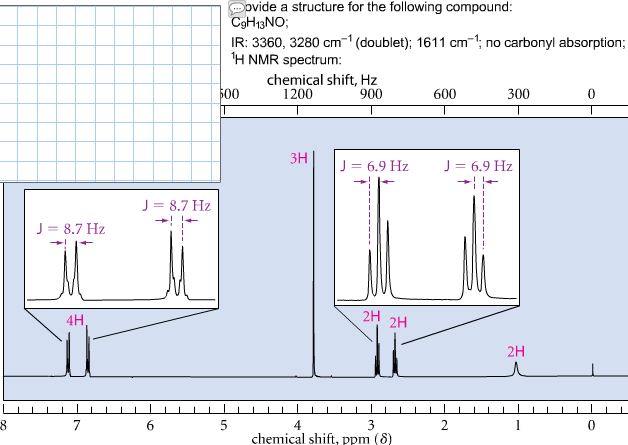# Problem: Provide a structure for the compound C9H13NO. IR:3360, 3280cm-1 (doublet); 1611 cm-1; no carbonyl absorption; 1H NMR spectrum:

###### FREE Expert Solution

Analyze the data given:

C9H13NO: Calculate IHD from formula:

For a given molecular formula, the IHD is calculated using:

$\overline{){\mathbf{IHD}}{\mathbf{=}}\frac{\left[2n+2\right]\mathbf{-}\mathbf{H}}{\mathbf{2}}}$

where n = number of carbons and H is:

+1 for hydrogen (H) or halogens (X)

0 for oxygen (O)

–1 for nitrogen (N)

79% (361 ratings)###### Problem Details

Provide a structure for the compound C9H13NO. IR:3360, 3280cm-1 (doublet); 1611 cm-1; no carbonyl absorption; 1H NMR spectrum: